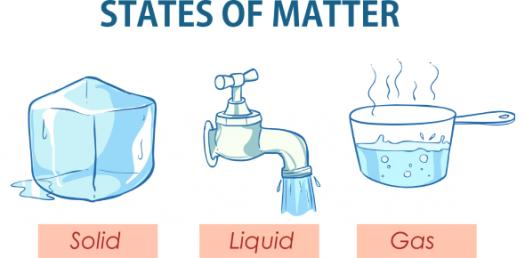# The Ultimate Science Test: Matter

46 Questions | Total Attempts: 5017Settings. Hey, how much do you already know about the matter, its properties, states, etc? Well, this quiz will put your knowledge on the ultimate test. It has essential to intermediate level of questions which will not just test you on the same but will also be a study session as well. So, let's start it here.

Related Topics
• 1.
• A.

Mass

• B.

Volume

• C.

Matter

• D.

Density

• 2.
The amount of matter in an object:
• A.

Mass

• B.

Volume

• C.

Matter

• D.

Density

• 3.
The amount of space an object takes up:
• A.

Mass

• B.

Volume

• C.

Matter

• D.

Density

• 4.
The mass per volume of a substance:
• A.

Mass

• B.

Volume

• C.

Matter

• D.

Density

• 5.
• A.

Ruler

• B.

• C.

Scale

• D.

Tripple Beam Balance

• 6.
The base unit used to measure Mass is:
• A.

Gram

• B.

Liter

• C.

Meter

• D.

Centimeter

• 7.
What is the equation used to measure the Volume of Regular Objects?
• A.

Weight x Length x Width

• B.

Base x Height x Length

• C.

Lenght x Width x Height

• D.

Mass x Weight x Length

• 8.
• A.

Cubic Centimeters (c3)

• B.

Cubic Litres (L3)

• C.

Cubic Grams (g3)

• D.

Cubic Meters (m3)

• 9.
What do you use to measure the Volume of Irregular Objects?
• A.

Ruler

• B.

Scale

• C.

• D.

Tripple Beam Balance

• 10.
• A.

Grams

• B.

Meters

• C.

Litres

• D.

Mililitres

• 11.
What is the unit of measurement used on a Graduated Cylinder?
• A.

Grams

• B.

Meters

• C.

Litres

• D.

Mililitres

• 12.
The particles found in Solids are close together.
• A.

True

• B.

False

• 13.
The particles found in Gases are close together.
• A.

True

• B.

False

• 14.
The particles found in Liquids are closer together than those found in Gases.
• A.

True

• B.

False

• 15.
Solids have the least amount of particles per volume.
• A.

True

• B.

False

• 16.
Gases have the least amount of particles per volume.
• A.

True

• B.

False

• 17.
• A.

1.0 ml/g

• B.

1.0 g/cm

• C.

1.0 g/ml

• D.

1.0 cm/g

• 18.
What is the smallest part of Matter (aka building block of matter)?
• A.

Proton

• B.

Neutron

• C.

Atom

• D.

Electron

• 19.
This is the center of every Atom:
• A.

Proton

• B.

Nucleus

• C.

Neutron

• D.

Electron

• 20.
This is the Positive Charge Particle in an Atom:
• A.

Proton

• B.

Nucleus

• C.

Neutron

• D.

Electron

• 21.
This is the Neutral/No-Charge Charge Particle in an Atom:
• A.

Proton

• B.

Nucleus

• C.

Neutron

• D.

Electron

• 22.
This is the Negative Charge Particle in an Atom:
• A.

Proton

• B.

Nucleus

• C.

Neutron

• D.

Electron

• 23.
Matter made of only 1 type of atom:
• A.

Compound

• B.

Element

• C.

Molecule

• D.

Substance

• 24.
This is the organized chart of elements:
• A.

Periodic Table of Elements

• B.

Periodic Chart of Elements

• C.

Periodic Graph of Elements

• D.

Periodic Chapter of Elements

• 25.
On a Periodic Table of Elements, what happens as you move across (left to right) each row?
• A.

The Atomic # increases

• B.

The Atomic # decreases

• C.

The Nucleaic # increases

• D.

The Nucleaic # decreases

• 26.
In a Periodic Table of Elements, the Elements in each column have:
• A.

Similar weight and mass

• B.

Similar properties and characteristics

• C.

Similar volume and density

• D.

Similar mass and density

• 27.
The number of Protons in and Atom:
• A.

Atomic Protons

• B.

Atomic Number

• C.

Atomic Mass

• D.

Atomic Neutrons

• 28.
The number of Protons and Neutrons in a Nucleus:
• A.

Atomic Protons

• B.

Atomic Number

• C.

Atomic Mass

• D.

Atomic Neutrons

• 29.
Two or more atoms joined together to make a new substance:
• A.

Substance

• B.

Molecule

• C.

Element

• D.

Compound

• 30.
The smallest part of a compound:
• A.

Sunstance

• B.

Molecule

• C.

Element

• D.

Compound

• 31.
• 32.
What is the Element represented by the symbol He?
• 33.
What is the element represented by the symbol C?
• 34.
• 35.
What is the Element represented by the symbol N?
• 36.
• 37.
• 38.
What is the element represented by the symbol Fe?
• 39.
What is the element represented by the symbol F?
• 40.
What is the Element represented by the symbol Ca?
• 41.
What is the Element represented by the symbol Al?
• 42.
The combination of 2 or more substances:
• A.

Solution

• B.

Mixture

• C.

Compund

• D.

Molecule

• 43.
A mixture in which all the particles are mixed evenly:
• A.

Solution

• B.

Mixture

• C.

Compund

• D.

Molecule

• 44.
To physically change the appearance of something:
• A.

Physical Change

• B.

Appearance Change

• C.

Chemical Change

• D.

Conservation of Matter

• 45.
The change in a substance that makes it something new:
• A.

Physical Change

• B.

Appearance Change

• C.

Chemical Change

• D.

Conservation of Matter

• 46.
During a chemical reaction, matter is neither gained or lost. It changes form. It is called:
• A.

Physical Change

• B.

Appearance Change

• C.

Chemical Change

• D.

Conservation of Matter